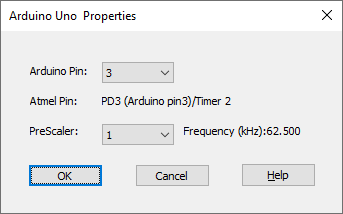#### PWM for Arduino

Target Category: Arduino

Target Sub-Category: PWM

Description: The PWM block can be used for several tasks including dimming an LED, generating modulated and audio signals, and providing variable speed motor control. The PWM block accepts scaled integer (fixed point) 8.16 input.Arduino Pin: Indicates the pin number on the Arduino board. Click here for Arduino pin mapping.

Atmel Pin: Indicates the timer associated with the selected PWM pin. Timers 0 and 2 are 8-bit timers; Timer 1 is a 16-bit timer. This is a read-only parameter.

PreScaler: Indicates the prescaler value used to divide the system clock frequency that drives the PWM counter. The frequency is the effective PWM waveform frequency, which is the system clock divided by the prescale value divided by 256 (period register size). Click here for the Arduino frequency table.